# Computer Graphics – 3D Rotation Transformations

• Last Updated : 14 Feb, 2021

Rotation in 3D is more nuanced as compared to the rotation transformation in 2D, as in 3D rotation we have to deal with 3-axes (x, y, z).There are three kinds of arbitrary rotation, here we can rotate an object just parallel(or along) a specific axis so that the coordinate about which the object rotates, remains unchanged and the rest two of the coordinates get changes. There are three kinds of such rotations that are possible:

1) Rotation about the x-axis: In this kind of rotation, the object is rotated parallel to the x-axis (principal axis), where the x coordinate remains unchanged and the rest of the two coordinates y and z only change.  Consider a point with initial coordinate P(x,y,z) in 3D space is made to rotate parallel to the principal axis(x-axis). The coordinate position would change to P'(x,y,z).

A rotation transformation matrix is used to calculate the new position coordinate P’, which shown as below: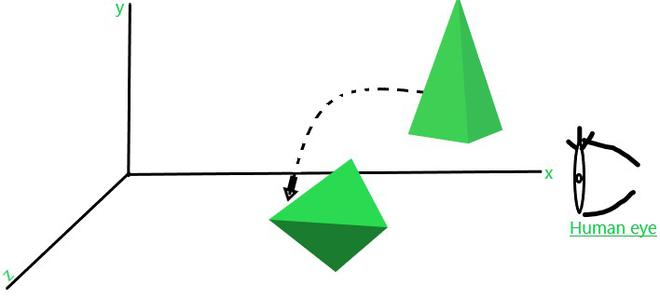Rotation along x-axis

2) Rotation about the y-axis: In this kind of rotation, the object is rotated parallel to the y-axis (principal axis), where the y coordinate remains unchanged and the rest of the two coordinates x and z only change.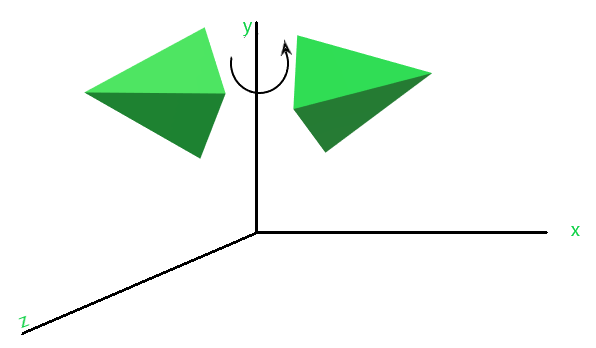Rotation along y-axis

Consider a point with initial coordinate P(x,y,z) in 3D space is made to rotate parallel to the principal axis(y-axis). The coordinate position would change to P'(x,y,z).3) Rotation about the z-axis: In this kind of rotation, the object is rotated parallel to the z-axis (principal axis), where the z coordinate remains unchanged and the rest of the two coordinates x and y only change.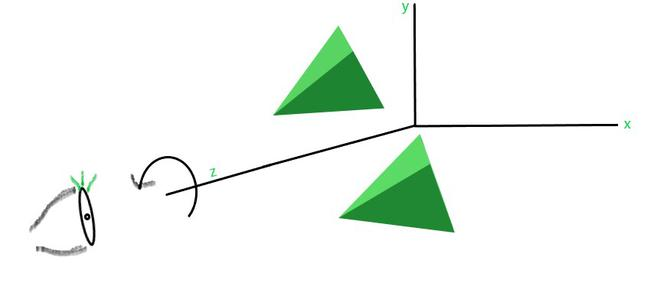Rotation along z-axis

Consider a point with initial coordinate P(x,y,z) in 3D space is made to rotate parallel to the principal axis(y-axis). The coordinate position would change to P'(x,y,z).Note: Rotation angle in Counter-clockwise direction is always considered +ve, while in the clockwise direction rotation angle is always considered -ve. It is a common convention that is always followed in 3D rotation.

Perform Rotation transformation over a cube ‘OABCDEFG’ and rotate it through 45* in the anticlockwise direction about the y-axis.

We are given with the following figure: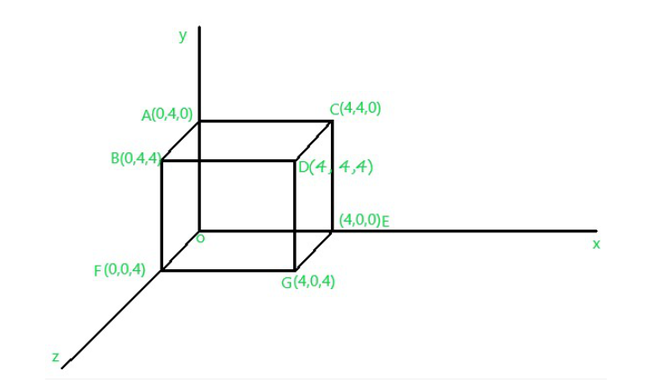Fig.1

Now, We’ll apply Rotational transformation along(parallel to) y-axis which is:Coordinate O after applying Rotational transformation, becomes:Coordinate A after applying Rotational transformation, becomes:Coordinate B after applying Rotational transformation, becomes:Coordinate C after applying Rotational transformation, becomes:Coordinate D after applying Rotational transformation, becomes:Coordinate E after applying Rotational transformation, becomes:Coordinate F after applying Rotational transformation, becomes:Coordinate G after applying Rotational transformation, becomes:So, the resultant cube that we get after successfully performing Rotational transformation on the cube ‘OABCDEF’ will look like: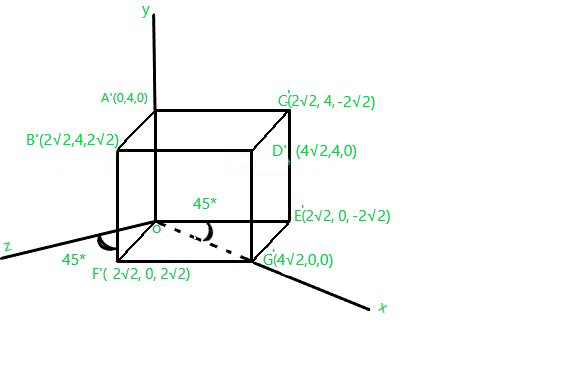My Personal Notes arrow_drop_up